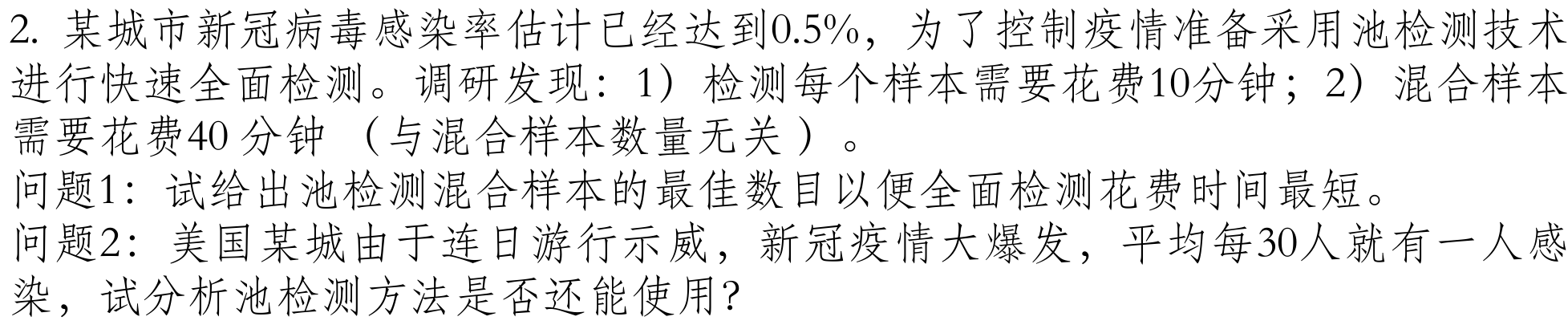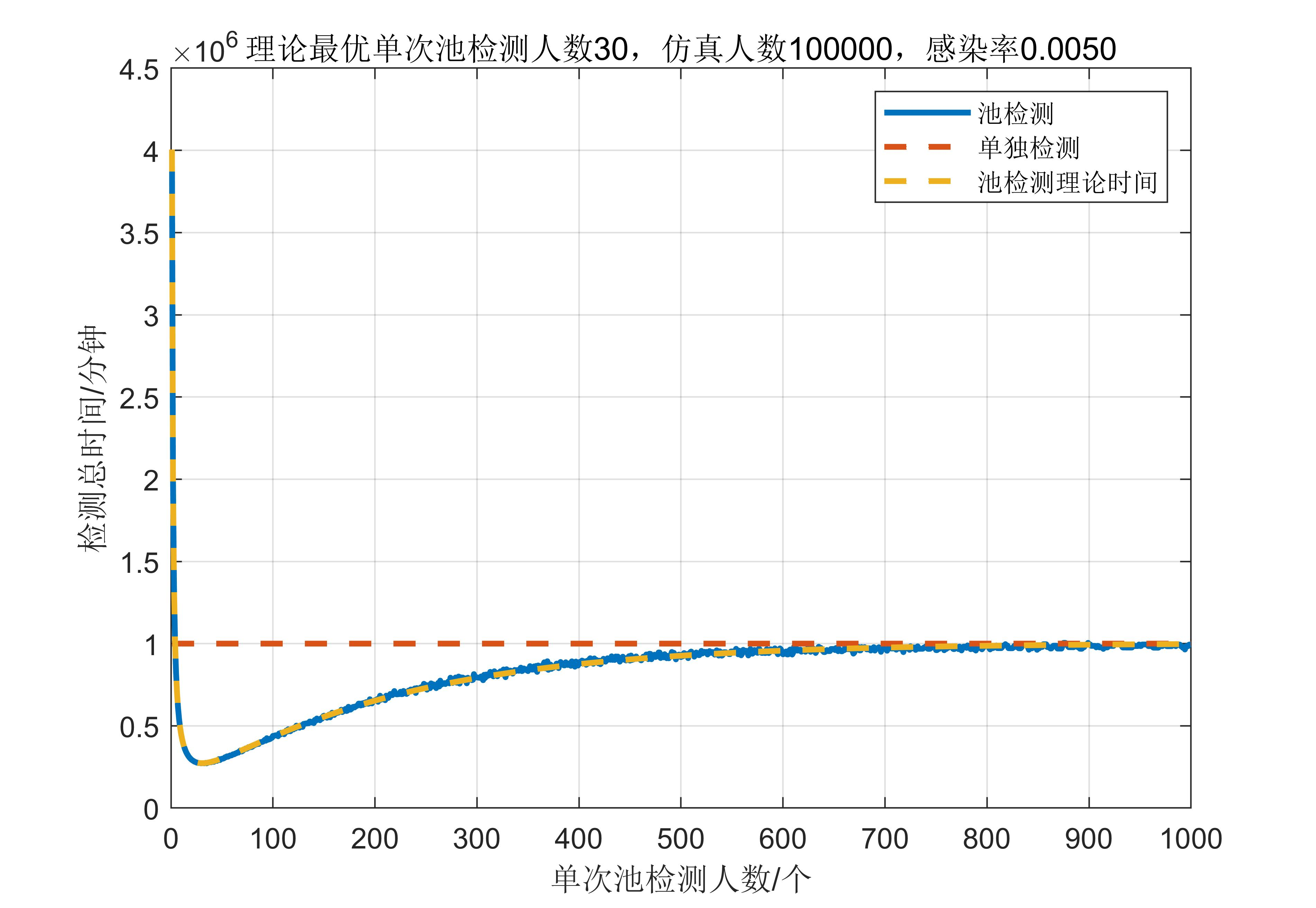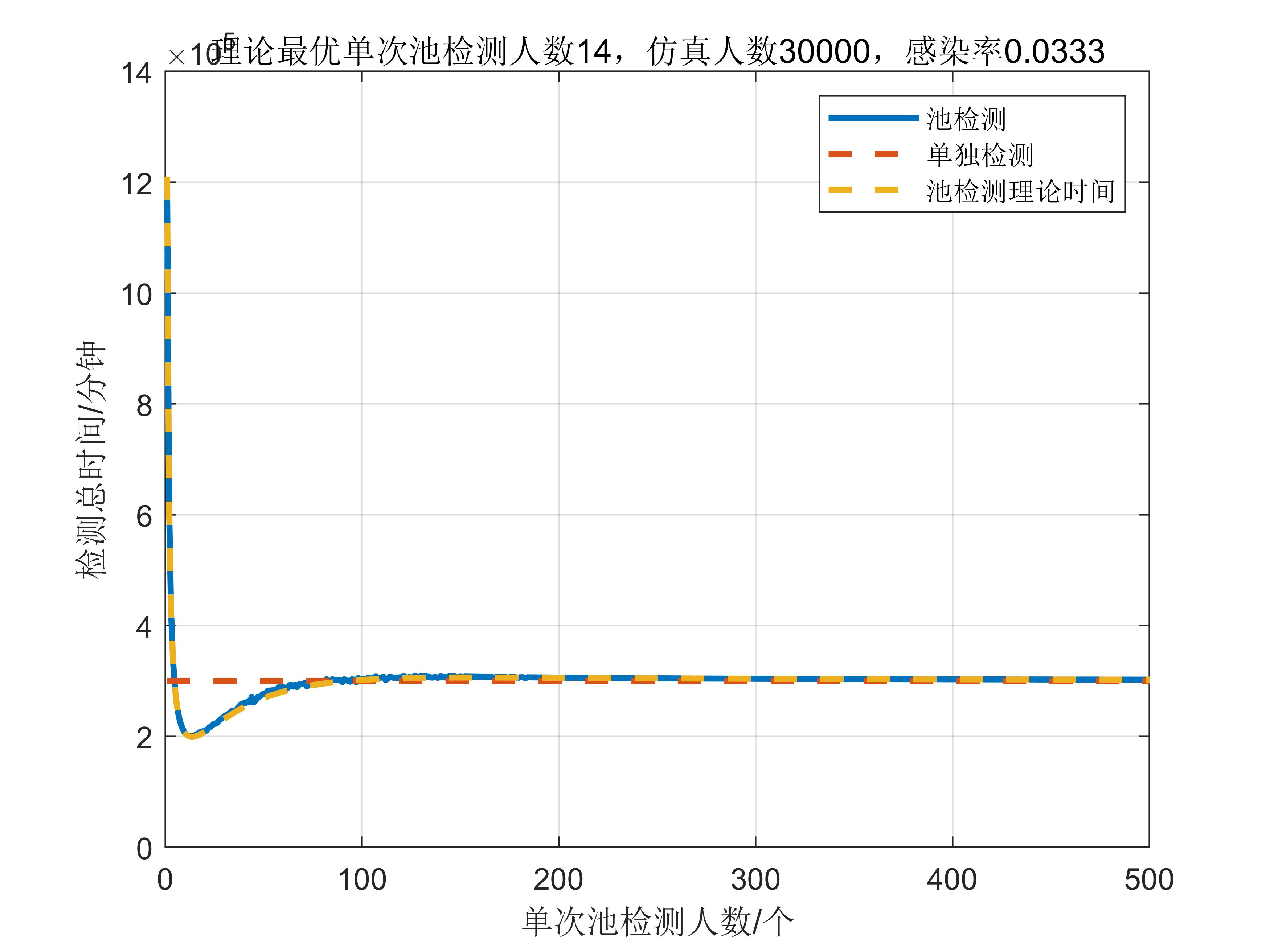# 新冠感染池检测选取最优池检测人数（概率模型理论公式+蒙特卡洛matlab仿真）数学建模 同时被 2 个专栏收录3 篇文章 0 订阅matlab代码如下:

%蒙特卡洛仿真新冠感染池检测选取最优池检测人数
%仿真时长约十几秒
N=100000;%仿真总人数
% N=30000;%仿真总人数
T_single=10;%单独检测时间
T_all=40;%群体检测时间
P_infect=0.005;%感染率
% P_infect=1/30;%感染率
N_infect=N*P_infect;%感染人数，需要是个整数
T0=N*T_single;%单独检测总时间
x=sparse(zeros(N,1));%模拟每个人，感染为1，没感染为0，用稀疏矩阵储存

for i=1:N_infect
while(1)%循环，随机让一个人感染
index=randi(N);%产生一个随机数
if x(index)==1%该人已感染，换一个
continue;
end
break;
end
x(index)=1;%感染
end

all_count=1:1000;%一次混合检测样本数量
T=[];
for j=1:size(all_count,2)%对每一个混合检测样本研究一下
i=1;
T(j)=0;%初始化时间
while(1)
iend=i+all_count(j)-1;%一段区间的最后
if iend>N%超出总人数了
iend=N;
end
infectnum=sum(x(i:iend));%池检测一次感染人数
T(j)=T(j)+T_all;%加上一次群体检测时间
if infectnum>0%有一人感染
T(j)=T(j)+T_single*(iend-i+1);%如果有人感染，需要对组内全部做单独检测
end
if iend==N%检测完了，结束
break;
end
i=i+all_count(j);%区间向后
end
end

F_T_theory=@(n) (T_all.*(1-P_infect).^n+(T_single*n+T_all).*(1-(1-P_infect).^n))./n;%理论计算公式
T_theory=N.*F_T_theory(all_count);%计算理论时间

plot(all_count,T,'linewidth',2);
hold on
plot(all_count,ones(size(all_count,2),1).*T0,'--','linewidth',2);
plot(all_count,T_theory,'--','linewidth',2);
xlabel("单次池检测人数/个")
ylabel("检测总时间/分钟")
legend("池检测","单独检测","池检测理论时间")
grid on
mincount=all_count(T==min(T))
mincount_theory=all_count(T_theory==min(T_theory))
title(sprintf("理论最优单次池检测人数%d，仿真人数%d，感染率%.4f",mincount_theory,N,P_infect))08-0603-04
02-10
04-22
07-064万+
08-28
01-23
09-07
01-302万+
11-28503
04-223855
12-013812
04-213494
12-023258
04-212559
11-292300
12-032210
11-252102
05-191983点击重新获取扫码支付余额充值